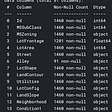# Table of Content

1. Installing NumPy
2. Importing Library
3. Introducing Array
4. Indexing
5. Some Inbuilt Functions
6. Reference Type
7. Random Distribution

# Installing NumPy

`pip install numpy`
`# Best practice, use an environment rather than install in the base envconda create -n my-envconda activate my-env# If you want to install from conda-forgeconda config --env --add channels conda-forge# The actual install commandconda install numpy`

# Importing Library

`import numpy as np`

# Introducing Array

`my_lst = [1,2,3,4,5]arr = np.array(my_lst)`
`type(arr)`
`arr`
`arr.shape`
`my_lst1=[1,2,3,4,5]my_lst2=[2,3,4,5,6]my_lst3=[9,7,6,8,9]arr=np.array([my_lst1,my_lst2,my_lst3])arr`
`arr.shape`
`arr.reshape(5,3)`

# Indexing

`arr=np.array([1,2,3,4,5,6,7,8,9])arr`
`my_lst1=[1,2,3,4,5]my_lst2=[2,3,4,5,6]my_lst3=[9,7,6,8,9]arr=np.array([my_lst1,my_lst2,my_lst3])`
`## arr[<row_start>:<row_end+1>,<col_start>:<col_end+1>,<step>]arr[1:,3:]`

# Some Inbuilt Functions

`arr=np.arange(0,10)arr`
`np.linspace(1,10,50)`

# Reference Type

`arr[3:]=100arr`
`array([ 0, 1, 2, 100, 100, 100, 100, 100, 100, 100])`
`arr1=arrarr1[3:]=500print(arr1)print(arr)`
`[  0   1   2 500 500 500 500 500 500 500][  0   1   2 500 500 500 500 500 500 500]`
`arr1=arr.copy()print(arr)arr1[3:]=1000print(arr1)`
`[   0    1    2 1000 1000 1000 1000 1000 1000 1000][  0   1   2 500 500 500 500 500 500 500]`
`val=2arr<2`
`arr[arr<2]`
`np.ones(4)`
`np.ones(4,dtype=int)`
`np.ones((2,5),dtype=float)`

# Random Distribution

`np.random.rand(3,3)`
`arr_ex=np.random.randn(4,4)arr_ex`
`np.random.randint(0,100,8)`
`np.random.random_sample((1,5))`

--

--

--

## More from Pavini Jain

Student at Jaypee Institute of Information Technology

Love podcasts or audiobooks? Learn on the go with our new app.

## Bouquet Analytics REST API —The Case for Self Governed User Management## Object Oriented Programming with C## Becoming a Butterfly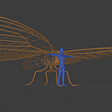## Chapter 16 -Collections and Generics: Data Structures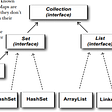## How To Create A VPS In AWS The Easy Way With Amazon Lightsail## Journaling Your Bugs For Fun And Profit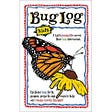## Combining constraints in PureScript## The Sixth Stage of Debugging## Pavini Jain

Student at Jaypee Institute of Information Technology

## Recognizing Handwritten Digits with Scikit-Learn## Data Types in Machine Learning## KNN — K-Nearest Neighbours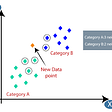## Predicting House Prices using Supervised Learning Methods